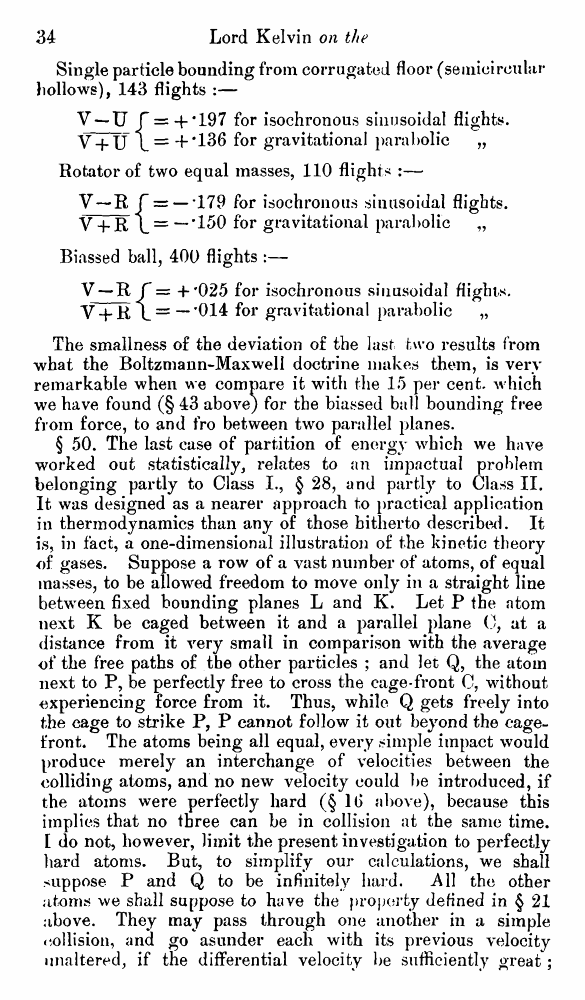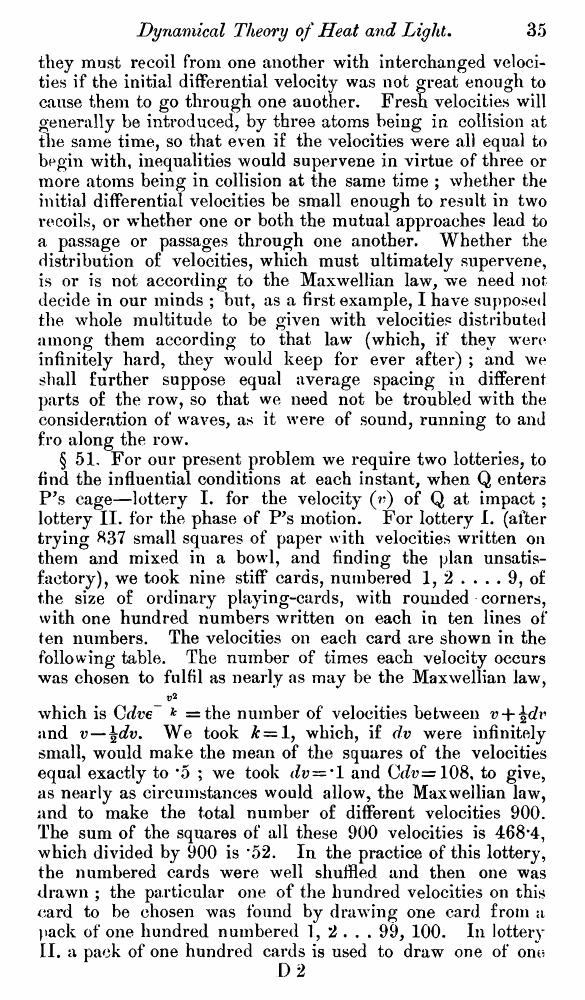# Lord Kelvin. Nineteenth-Century Clouds over the Dynamical Theory of Heat and Light. // Phil. Mag. S. 6. Vol. 2. No. 7. July 1901.

В начало   Другие форматы   <<<     Страница 34   >>>1  2  3  4  5  6  7  8  9  10  11  12  13  14  15  16  17  18  19  20  21  22  23  24  25  26  27  28  29  30  31  32  33  34 35  36  37  38  39  40 Single particle bounding from corrugated floor (semicircular hollows), 143 flights :— V —U f = +*197 for isochronous sinusoidal flights. V + U L = +‘136 for gravitational parabolic „ Rotator of two equal masses, 110 flights :— V—R f =r —'179 for isochronous sinusoidal flights. V + R \ = “*-’150 for gravitational parabolic „ Biassed ball, 400 flights :— V — R f = + '025 for isochronous sinusoidal flights. V + R \ = —’014 for gravitational parabolic „ The smallness o£ the deviation of the last two results from what the Boltzmann-Maxwell doctrine makes them, is very remarkable when we compare it with the 15 per cent, which we have found (§ 43 above) for the biassed ball bounding free from force, to and fro between two parallel planes. § 50. The last case of partition of energy which we have worked out statistically, relates to an impactual problem belonging partly to Class I., § 28, and partly to Class II. It was designed as a nearer approach to practical application in thermodynamics than any of those hitherto described. It is, in fact, a one-dimensional illustration of the kinetic theory of gases. Suppose a row of a vast number of atoms, of equal masses, to be allowed freedom to move only in a straight line between, fixed bounding planes L and K. Let P the atom next K be caged between it and a parallel plane 0, at a distance from it very small in comparison with the average of the free paths of the other particles ; and let Q, the atom next to P, be perfectly free to cross the cage-front C, without experiencing force from it. Thus, while Q gets freely into the cage to strike P, P cannot follow it out beyond the cage-front. The atoms being all equal, every simple impact would produce merely an interchange of velocities between the colliding atoms, and no new velocity could be introduced, if the atoms were perfectly hard (§ 1 i> above), because this implies that no three can be in collision at the same time. [ do not, however, limit the present investigation to perfectly hard atoms. But, to simplify our calculations, we shall suppose P and Q to be infinitely hard. All the other atoms we shall suppose to have the property defined in § 21 above. They may pass through one another in a simple collision, and go asunder each with its previous velocity unaltered, if the differential velocity be sufficiently great; they must recoil from one another with interchanged velocities if the initial differential velocity was not great enough to cause them to go through one auother. Fresh velocities will generally be introduced, by three atoms being in collision at the same time, so that even if the velocities were all equal to begin with, inequalities would supervene in virtue of three or more atoms being in collision at the same time ; whether the initial differential velocities be small enough to result in two recoils, or w’hether one or both the mutual approaches lead to a passage or passages through one another. Whether the distribution of velocities, which must ultimately supervene, is or is not according to the Maxwellian law, we need not decide in our minds ; but, as a first example, I have supposed the whole multitude to be given with velocities distributed among them according to that law (which, if they were infinitely hard, they would keep for ever after) ; and we shall further suppose equal average spacing in different parts of the row, so that we need not be troubled with the consideration of waves, as it were of sound, running to and fro along the row. § 51, For our present problem we require two lotteries, to find the influential conditions at each instant, when Q enters P’s cage—lottery I. for the velocity (r) of Q at impact ; lottery II. for the phase of P’s motion. For lottery I. (after trying 837 small squares of paper with velocities written on them and mixed in a bowl, and finding the plan unsatisfactory), we took nine stiff cards, numbered 1, 2 .... 9, of the size of ordinary playing-cards, with rounded corners, with one hundred numbers written on each in ten lines of* ten numbers. The velocities on each card are shown in the following table. The number of times each velocity occurs was chosen to fulfil as nearly as may be the Maxwellian law, which is Cdve~ k =the number of velocities between v + and 17—\dv. We took £ = 1, which, if dv were infinitely small, would make the mean of the squares of the velocities equal exactly to *5 ; we took dv = ' 1 and Crfv=108, to give, as nearly as circumstances would allow, the Maxwellian law, and to make the total number of different velocities 900. The sum of the squares of all these 900 velocities is 468*4, which divided by 900 is *52. In the practice of this lottery, the numbered cards were well shuffled and then one was drawn ; the particular one of the hundred velocities on this card to be chosen was found by drawing one card from a pack of one hundred numbered 1, 2 . . . 99, 100. In lottery II. a pack of one hundred cards is used to draw one of onr? D 2